# Fall 2021 Final Exam

Instructor(s): Justin Eldridge

This exam was administered online. The exam was open-notes, open-book. Students had 180 minutes to take this exam.

Welcome to the Final Exam for DSC 80. This is an open-book, open-note exam, but you are not permitted to discuss the exam with anyone until after grades are released.

This exam has several coding questions. These questions will be manually graded, and your answers are not expected to be syntactically-perfect! Be careful not to spend too much time perfecting your code.

## Problem 1

This table below contains energy usage information for every building owned and managed by the New York City Department of Citywide Administrative Services (DCAS). DCAS is the arm of the New York City municipal government which handles ownership and management of the city’s office facilities and real estate inventory. The organization voluntarily publicly discloses self-measured information about the energy use of its buildings.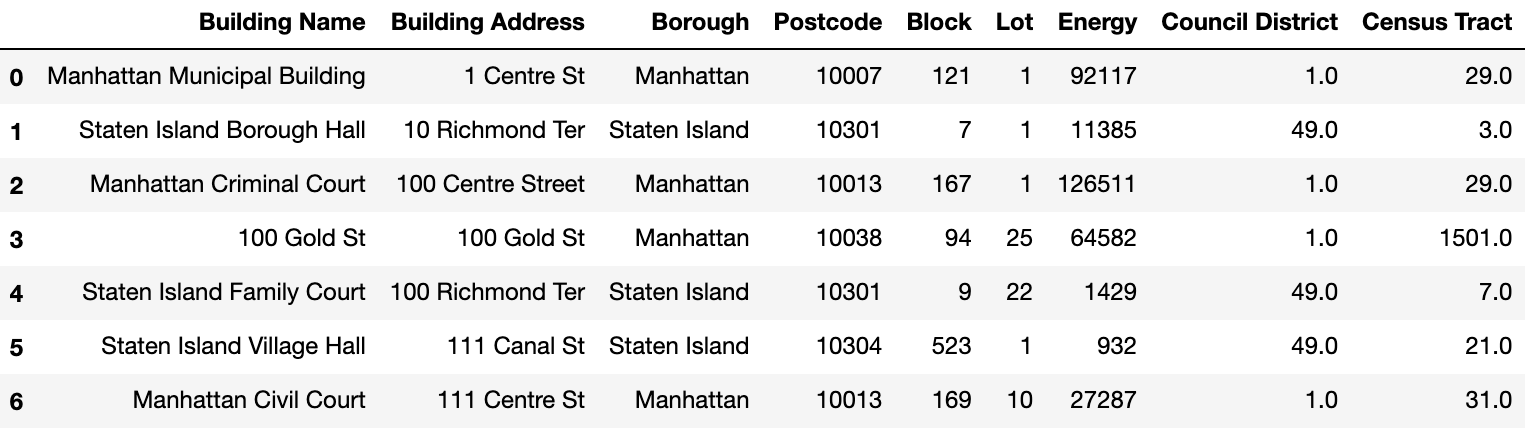Assume that the table has been read into the variable named df. The result of df.info() is shown below: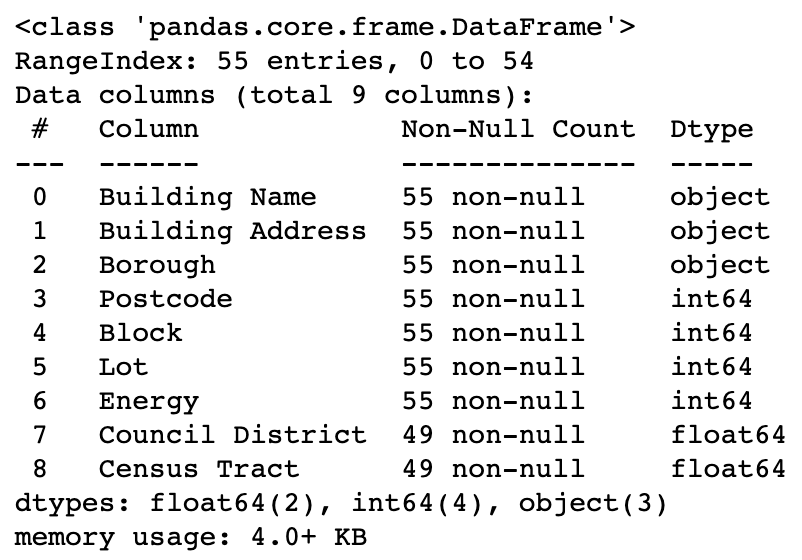### Problem 1.1

Write a piece of code that computes the name of the borough whose median building energy usage is the highest.

Note: your code will be graded manually, and it is not expected to be perfect. Be careful to not spend too much time trying to make your code perfect!

Answer: df.groupby('Borough')['Energy'].median().idxmax()

The idea is to perform a groupby and get the median within each group and then somehow get the group with the largest median.

##### Difficulty: ⭐️

The average score on this problem was 91%.

### Problem 1.2

Write a line of code to normalize the building addresses. To normalize an address, replace all spaces with underscores, change “Street” to “St”, change “Avenue” to “Av”, and convert to lower case. Your code should evaluate to a series containing the normalized addresses.

Answer: df['Building Addresss'].apply(lambda x: x.replace(' ', '_').replace('Avenue', 'Av').replace('Street', 'St').lower())

##### Difficulty: ⭐️

The average score on this problem was 90%.

### Problem 1.3

From the table above, it looks like it is common for city buildings to have addresses that start with the number ‘1’; e.g., “100 Centre Street”. Write a piece of code that plots a histogram showing the number of times each number appears as the first number in an address.

Answer: df['Building Addresss'].apply(lambda x: int(x)).plot(kind = 'hist')

The apply part of the code gets the first number of each address, and then plots the resulting Series as a histogram.

##### Difficulty: ⭐️

The average score on this problem was 90%.

### Problem 1.4

Suppose the following code is run:

from sklearn.preprocessing import OneHotEncoder
oh = OneHotEncoder()
oh.fit_transform(df[['Borough']]).mean(axis=0)

What will be the result?

• An array of size 5 containing the proportion of buildings in each of the five boroughs.

• An array with as many entries as there are buildings containing 0.2 in each spot.

• An array of size 5 containing all zeros.

• An array of size 5 containing one 1 and the rest zeros.

One-Hot-Encoding will create 5 columns representing each ‘Borough’. For each building, a 1 will be filled in the column of the ‘Borough’ in which the building belongs to, and 0 for the other 4 columns. Thus taking the mean of each of the 5 ‘Borough’ columns will just produce the proportion of buildings in each of the 5 boroughs, yielding Option A.

##### Difficulty: ⭐️

The average score on this problem was 90%.

### Problem 1.5

Write a piece of code (perhaps more than one line) that computes the proportion of addresses in each borough that end with either St or Street.

def match(x):
return (x.endswith('St')) | (x.endswith('Street'))

df.groupby('Borough')['Building Address'].mean()

##### Difficulty: ⭐️⭐️

The average score on this problem was 79%.

## Problem 2

For this problem, consider the HTML document shown below:

<html>
<title>Data Science Courses</title>

<body>
<h1>Welcome to the World of Data Science!</h1>

<h2>Current Courses</h2>

<div class="course_list">

<img alt="Course Banner", src="courses.png">
<p>
Here are the courses available to take:
</p>
<ul>
<li>Machine Learning</li>
<li>Design of Experiments</li>
</ul>

<p>
For last quarter's classes, see <a href="./2021-sp.html">here</a>.
</p>

</div>

<h2>News</h2>

<div class="news">
<p class="news">
New course on <b>Visualization</b> is launched.
See <a href="https://http.cat/301.png" target="_blank">here</a>
</p>
</div>

</body>
</html>

### Problem 2.1

How many children does the div node with class course_list contain in the Document Object Model (DOM)?

Looking at the code, we could see that the div with class course_list has 4 children, namely: a img node, p node, ul node and p node.

##### Difficulty: ⭐️⭐️

The average score on this problem was 78%.

### Problem 2.2

Suppose the HTML document has been parsed using doc = bs4.BeautifulSoup(html). Write a line of code to get the h1 header text in the form of a string.

Answer: doc.find('h1').text

Since there’s only one h1 element in the html code, we could simply do doc.find('h1') to get the h1 element. Then simply adding .text will get the text of the h1 element in the form of a string.

##### Difficulty: ⭐️

The average score on this problem was 93%.

### Problem 2.3

Suppose the HTML document has been parsed using doc = bs4.BeautifulSoup(html). Write a piece of code that scrapes the course names from the HTML. The value returned by your code should be a list of strings.

Answer: [x.text for x in doc.find_all('li')]

Doing doc.find_all('li') will find all li elements and return it is the form of a list. Simply performing some basic list comprehension combined .text to get the text of each li element will yield the desired result.

##### Difficulty: ⭐️

The average score on this problem was 93%.

### Problem 2.4

There are two links in the document. Which of the following will return the URL of the link contained in the div with class news? Mark all that apply.

• doc.find_all('a').attrs['href']

• doc.find('a').attrs['href']

• doc.find(a, class='news').attrs['href']

• doc.find('div', attrs={'class': 'news'}).find('a').attrs['href']

• doc.find('href', attrs={'class': 'news'})

Answer: Option A and Option D

• Option A: This option works because doc.find_all('a') will return a list of all the a elements in the order that it appears in the HTML document, and since the a with class news is the second a element appearing in the HTML doc, we do  to select it (as we would in any other list). Finally, we return the URL of the a element by getting the 'href' attribute using .attrs['href']
• Option B: This does not work because .find will only find the first instance of a, which is not the one we’re looking for.
• Option C: This does not work because there are no quotations around the a.
• Option D: This option works becuase doc.find('div', attrs={'class': 'news'}) will first find the div element with class='news', and then find the a element within that element and get the href attribute of that, which is what we want.
• Option E: This does not work because there is no href element in the HTML document.

##### Difficulty: ⭐️

The average score on this problem was 90%.

### Problem 2.5

What is the purpose of the alt attribute in the img tag?

• It provides an alternative image that will be shown to some users at random

• It creates a link with text present in alt

• It provides the text to be shown below the image as a caption

• It provides text that should be shown in the case that the image cannot be displayed

^pretty self-explanatory.

##### Difficulty: ⭐️

The average score on this problem was 98%.

### Problem 2.6

You are scraping a web page using the requests module. Your code works fine and returns the desired result, but suddenly you find that when you run your code it starts but never finishes – it does not raise an error or return anything. What is the most likely cause of the issue?

• The page has a very large GIF that hasn’t stopped playing

• You have made too many requests to the server in too short of a time, and you are being “timed out”

• The page contains a Unicode character that requests cannot parse

• The page has suddenly changed and has caused requests to enter an infinite loop

• Option A: We can pretty confidentely (I hope) rule this option out since whether or not a GIF has stopped playing or not shouldn’t affect our web scraping.
• Option B: This answer is right because a server will time you out (and potentially block you) if you make too many requests to the server.
• Option C: This shouldn’t cause your code to never finish, rather, it’s more likely that the request module just doesn’t process said Unicode character correctly or it throws an error.
• Option D: Again, this shouldn’t cause your code to never finish, rather, the request module will just parse the older version of the website at the time you called it.

##### Difficulty: ⭐️⭐️

The average score on this problem was 78%.

## Problem 3

### Problem 3.1

You are creating a new programming language called IDK. In this language, all variable names must satisfy the following constraints:

• They must start with Um, Umm, Ummm, etc. That is, a capital U followed by one or more m’s.
• Following this may be any string of one or more letters. The first letter must be capitalized.
• The variable name must end with a single question mark.
• Numbers or other special characters are not allowed.

Examples of valid variable names: UmmmX?, UmTest?, UmmmmPendingQueue? Examples of invalid variable names: ummX?, Um?, Ummhello?, UmTest

Write a regular expression pattern string pat to validate variable names in this new language. Your pattern should work when re.match(pat, s) is called.

Answer: 'U(m+)[A-Z]([A-z]*)(\?)'

Starting our regular expression, it’s not too difficult to see that we need a 'U' followed by (m+) which will match with a singular capital 'U' followed by at least one lowercase 'm'. Next it is required that we follow that up with any string of letters, where the first letter is capitalized. we do this with '[A-Z]([A-z]*)', where '[A-Z]' will match with any capital letter and '([A-z]*)' will match with lowercase and uppercase letters 0 or more times. Finally we end the regex with '\?' which matches with a question mark.

##### Difficulty: ⭐️⭐️

The average score on this problem was 87%.

### Problem 3.2

Which of the following strings will be matched by the regex pattern \$\s*\d{1,3}(\.\d{2})?? Note that it should match the complete string given. Mark all that apply. •$ 100

• $10.12 •$1340.89

• $1456.8 •$478.23

• $.99 Answer: Option A, Option B and Option E Let’s dissect what the regex in the question actually means. First, the '\$' simply matches with any question mark. Next, '\s*' matches with whitespace characters 0 or more times, and '\d{1,3}' will match with any digits 1-3 times inclusive. Finally, '(\.\d{2})?' will match with any expression consisting of a period and any two digits following that period 0 or 1 times (due to the '?' mark). With those rules in mind, it’s not too difficult to check that Options A, B and E work.

Options C, D and F don’t work because none of those expressions have 1-3 digits before the period.

##### Difficulty: ⭐️⭐️

The average score on this problem was 83%.

### Problem 3.3

“borough”, “brough”, and “burgh” are all common suffixes among English towns. Write a single regular expression pattern string pat that will match any town name ending with one of these suffixes. Your pattern should work when re.match(pat, s) is called.

Answer: '(.*)((borough$)|(brough$)|(burgh$))' We will assume that the question wants us to create a regex that matches with any string that ends with the strings described in the problem. First, '(.*)' will match with anything 0 or more times at the start of the expression. Then '((borough$)|(brough$)|(burgh$))' will match with any of the described strings in the problem , since '\$' indicates the end of string and '|' is just or in regex.

##### Difficulty: ⭐️⭐️

The average score on this problem was 82%.

## Problem 4

For this problem, consider the following data set of product reviews.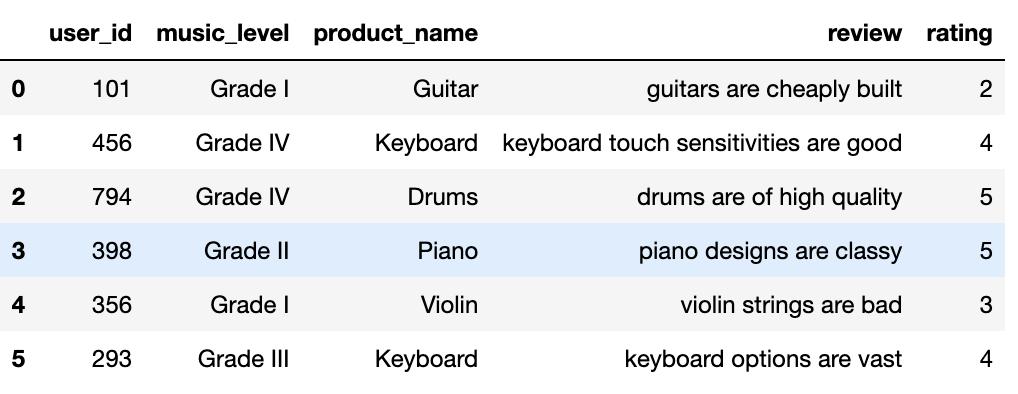### Problem 4.1

What is the Inverse Document Frequency (IDF) of the word “are” in the review column? Use base-10 log in your computations.

The IDF is computed by the logarithm of the quotient of total number of documents divided by number of documents with the said term in it. Since there are 6 documents and all 6 have the word “are” in it, we the IDF is just \log{(\frac{6}{6})} which is just 0.

##### Difficulty: ⭐️

The average score on this problem was 97%.

### Problem 4.2

Calculate the TF-IDF score of “keyboard” in the last review. Use the base-10 logarithm in your calculation.

TF is just the number of times a term appears in a document divided by the number of terms in a document. Thus the TF score of “keyboard” in the last review is just \frac{1}{4}

The IDF score is just log{(\frac{6}{2})}, since there are 2 documents with “keyboard” in it and documents total.

Thus multiplying \frac{1}{4} \times \log{(\frac{6}{2})} yields 0.119.

##### Difficulty: ⭐️⭐️

The average score on this problem was 81%.

### Problem 4.3

The music store wants to predict rating using other features in the dataset. Before that, we have to deal with the categorical variables - music_level, and product_name. How should you encode the music_level variable for the modeling?

• One-hot encoding

• Bag of words

• Ordinal encoding

• Tf-Idf

In ordinal encoding, each unique category is assigned an integer value. Thus it would make sense to encode music_level with Ordinal encoding since there an inherent order to music_level. i.e. ‘Grade IV’ would be “higher” than ‘Grade I’ in music_level.

Bag of words doesn’t work since we’re not necessarily trying to encode multi-worded strings, and TF-IDF simply doesn’t make sense for encoding. One-hot encoding doesn’t work as well in this case since music_level is an ordinal categorical variable.

##### Difficulty: ⭐️

The average score on this problem was 100%.

### Problem 4.4

How should you encode the product_name variable for the modeling?

• One-hot encoding

• Bag of words

• Ordinal encoding

• Tf-Idf

##### Difficulty: ⭐️

The average score on this problem was 98%.

Since product_name is a nominal categorical variable, it would make sense to encode it using One-hot encoding. Ordinal encoding wouldn’t work this time since product_name is not an ordinal categorical variable. We could eliminate the rest of the options from similar reasoning as the question above.

### Problem 4.5

True or False: the TF-IDF score of a term depends on the document containing it.

• True

• False

TF is calculated by the number of times a term appears in a document divided by the number of terms in the document, which depends on the document itself. So if TF depends on the document itself, so does the TF-IDF score.

##### Difficulty: ⭐️

The average score on this problem was 97%.

### Problem 4.6

Suppose you perform a one-hot encoding of a Series containing the following music genres:

[
"hip hop",
"country",
"polka",
"country",
"pop",
"pop",
"hip hop"
]

Assume that the encoding is created using OneHotEncoder(drop='first') transformer from sklearn.preprocessing (note the drop='first') keyword.

How many columns will the one-hot encoding table have?

Normal One-hot encoding will yield 4 columns, one for each of the following categories: “hip-hop”, “country”, “polka”, and “pop”. However, drop='first' will drop the first column after One-hot encoding which will yield 4 - 1 = 3 columns.

##### Difficulty: ⭐️⭐️

The average score on this problem was 80%.

## Problem 5

A credit card company wants to develop a fraud detector model which can flag transactions as fraudulent or not using a set of user and platform features. Note that fraud is a rare event (about 1 in 1000 transactions are fraudulent).

Suppose the model provided the following confusion matrix on the test set. Here, 1 (a positive result) indicates fraud and 0 (a negative result) indicates otherwise. Let’s evaluate it to understand the model performance better.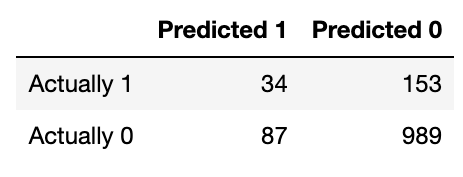Use this confusion matrix to answer the following questions.

### Problem 5.1

What is the accuracy of the model shown above? Your answer should be a number between 0 and 1.

Accuracy is just calculated as the number of correct predictions divided by total predictions. From the table, we could see that there are 34 + 989 = 1023 correct predictions, and 34 + 153 + 87 + 989 = 1263, giving us a 1023/1263 or 0.809 accuracy.

##### Difficulty: ⭐️

The average score on this problem was 94%.

### Problem 5.2

How many false negatives were produced by the model shown above?

A false negative is when the model predicts a negative result when the actual value is positive. So in the case of this model, a false negative would be when the model predicts 0 when the actual value should be 1. Simply looking at the table shows that there are 153 false negatives.

##### Difficulty: ⭐️

The average score on this problem was 91%.

### Problem 5.3

What is the precision of the model shown above?

The precision of the model is given by the number of True Positives (TP), divided by the sum of the number of True Positives (TP) and False Positives (FP) or \frac{TP}{TP + FP}. Plugging the appropriate values in the formula gives us 34/(34 + 87) = 34/121 or 0.281.

##### Difficulty: ⭐️⭐️

The average score on this problem was 85%.

### Problem 5.4

What is the recall of the model shown above?

The precision of the model is given by the number of True Positives (TP), divided by the sum of the number of True Positives (TP) and False Negatives (FN) or \frac{TP}{TP + FN}. Plugging the appropriate values in the formula gives us 34/(34 + 153) = 34/187 or 0.18.

##### Difficulty: ⭐️⭐️

The average score on this problem was 82%.

### Problem 5.5

What is the accuracy of a “naive” model that always predicts that the transaction is not fraudulent? Your answer should be a number between 0 and 1.

If our model always predicts not fraudulent or 0, then we will get an accuracy of \frac{87+989}{34+153+87+989} or 0.852

##### Difficulty: ⭐️⭐️

The average score on this problem was 78%.

### Problem 5.6

Describe a simple model that will achieve 100% recall despite it being a poor classifier.

Answer: A model that always guesses fraudulent.

Recall that the formula for recall (no pun intended) is given by \frac{TP}{TP + FN}. Thus we reason that if we want to achieve 100% recall, we should simply achieve no False Negatives (or FN = 0), since that will gives us \frac{TP}{TP + 0}, which is obviously just 1. Therefore, if we never guess 0 or non-fraudulent, we’ll never get any False Negatives (b/c getting any FN requires our model to predict negatives for some values). Hence a mmodel that always guesses fraudulent will achieve 100% recall, despite obviously being a bad model.

##### Difficulty: ⭐️⭐️

The average score on this problem was 86%.

### Problem 5.7

Suppose you consider false negatives to be more costly than false positives, where a “positive” prediction is one for fraud. Assuming that precision and recall are both high, which is better for your purposes:

• A model with higher recall than precision.

• A model with higher precision than recall.

Since we consider false negatives to be more costly, it would therefore make sense to favor on a model that puts more emphasis on recall because, again, the formula for recall is given by \frac{TP}{TP + FN}. O the other hand, precision doesn’t care about false negatives, so the answer here is Option A.

##### Difficulty: ⭐️⭐️

The average score on this problem was 85%.

## Problem 6

### Problem 6.1

Suppose you split a data set into a training set and a test set. You train your model on the training set and test it on the test set.

True or False: the training accuracy must be higher than the test accuracy.

• True

• False

There is no direct correlation between the training accuracy of a model and the test accuracy of the model, since the way you decide to split your data set is largely random. Thus there might be a possibility that your test accuracy ends up higher than your training accuracy. To illustrate this, suppose your training data consists of 100 data points and your test data consists of 1 data point. Now suppose your model achieves a training accuracy of 90%, and when you proceed to test it on the test set, your model predicts that singular data point correctly. Clearly youre test accuracy is now higher than your training accuracy.

##### Difficulty: ⭐️⭐️

The average score on this problem was 75%.

### Problem 6.2

Suppose you create a 70%/30% train/test split and train a decision tree classifier. You find that the training accuracy is much higher than the test accuracy (90% vs 60%). Which of the following is likely to help significantly improve the test accuracy? Select all that apply. You may assume that the classes are balanced.

• Reduce the number of features

• Increase the number of features

• Decrease the max depth parameter of the decision tree

• Increase the max depth parameter of the decision tree

Answer: Option A and Option C

When your training accuracy is significaly higher than your test accuracy, it tells you that your model is perhaps overfitting the data. And thus we wish to reduce the complexity of our model. From the choices given, we can reduce the complexity of our model by reducing the number of features or decreasing the max depth parameter of the decision tree. (Recall that the greater depth the a decision tree model, the more complex the model).

##### Difficulty: ⭐️⭐️⭐️

The average score on this problem was 65%.

### Problem 6.3

Suppose you are training a decision tree classifier as part of a pipeline with PCA. You will need to choose three parameters: the number of components to use in PCA, the maximum depth of the decision tree, and the minimum number of points needed for a leaf node. You’ll do this using sklearn’s GridSearchCV which performs a grid search with k-fold cross validation.

Suppose you’ll try 3 possibilities for the number of PCA parameters, 5 possibilities for the max depth of the tree, 10 possibilities for the number of points needed for a leaf node, and use k=5 folds for cross-validation.

How many times will the model be trained by GridSearchCV?

Note that our grid search will test every combination of hyperparameters for our model, which gives us a total of 3 * 5 * 10 = 150 combinations of hyperparameters. However, we all perform k-fold cross validation with 5 folds, meaning that our model is trained 5 times for each combination of hyperparameters, giving us a grand total of 5 * 150 = 750.

##### Difficulty: ⭐️⭐️

The average score on this problem was 84%.

### Problem 6.4

The plot below shows the distribution of reported gas mileage for two models of car.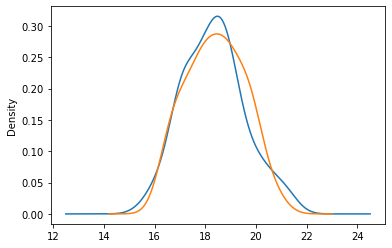What test statistic is the best choice for testing whether the two empirical distributions came from different underlying distributions?

• the TVD

• the absolute difference in means

• the signed difference in means

• the Kolmogorov-Smirnov Statistic

TVD simply doesn’t work here since we’re not working with categorical data. Any sort of difference in means here wouldn’t really tell us much either since both distributions seem to have basically the same mean. Thus to tell whether the two distributions are different, it would be best if we used K-S statistic.

##### Difficulty: ⭐️⭐️

The average score on this problem was 88%.

### Problem 6.5

Suppose 1000 people are surveyed. One of the questions asks for the person’s age. Upon reviewing the results of the survey, it is discovered that some of the ages are missing – these people did not respond with their age. What is the most likely type of this missingness?

• Missing At Random

• Missing Completely At Random

• Not Missing At Random

• Missing By Design

The most likely mechanism for this is NMAR becuase there is a good reason why the missingess depends on the values themselves, namely, if one is a baby (like 1 or 2 years old), theres a a high probablility that they literally just aren’t aware of their age and hence are less likely to answer. On the other end of the spectrum, older people might also refrain from answering their age.

MAR doesn’t work here because there aren’t really any other columns that’ll tell us anything about the likelihood of the missigness of the age column. MD clearly doesn’t work here since we have no way of determining the missing values through the other columns. Alghough MCAR could be a possibility, it is more approrpiate that this problem illustrates NMAR rather than MCAR.

##### Difficulty: ⭐️⭐️

The average score on this problem was 76%.

### Problem 6.6

Consider a data set consisting of the height of 1000 people. The data set contains two columns: height, and whether or not the person is an adult.

Suppose that some of the heights are missing. Among those whose heights are observed there are 400 adults and 400 children; among those whose height is missing, 50 are adults and 150 are children.

If the mean height is computed using only the observed data, which of the following will be true?

• the mean will be biased low

• the mean will be biased high

• the mean will be unbiased

Since there are more missing children heights than missing adult heights, (we will assume that adults are taller than children), the observed mean will be higher than the actual total mean, or the observed mean will be biased high.

##### Difficulty: ⭐️

The average score on this problem was 94%.

### Problem 6.7

We have built two models which have the following accuracies: Model 1: Train score: 93%, Test score: 67%. Model 2: Train score: 84%, Test score: 80% Which of the following model will you choose to use to make future predictions on unseen data? You may assume that the class labels are balanced.

• Model 1

• Model 2

When testing our models, we only really care about the test scores of our models, and so we’ll typically choose the model with the higher test score which is Model 2.

##### Difficulty: ⭐️

The average score on this problem was 99%.

### Problem 6.8

Suppose we retrain a decision tree model, each time increasing the max_depth parameter. As we do so, we plot the test error. What will we likely see?

• The test error will first decrease, then increase.

• The test error will decrease.

• The test error will first increase, then decrease.

• The test error will remain unchanged.

As we increase our max_depth parameter of our model, our model’s test error will decrease. It’ll keep decreasing until we’ve reached the optimal max_depth parameter of our model, for which after that the test error will start to increase (due to overfitting). Thus the correct answer is Option A.